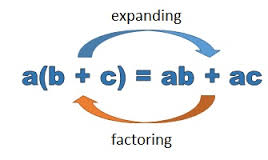Factoring Algebraic Expressions
3 years ago
priestmer
Save
Edit
Host a game
Live GameLive
Homework
Solo Practice
Practice11 QuestionsShow answers
• Question 1
120 seconds
Q. Factor
2x + 8
2(x + 8)
2(x + 4)
• Question 2
120 seconds
Q. Factor
10 + 15x
5(2 + 3x)
2(5 + 10x)
• Question 3
120 seconds
Q. True or False
12x - 8 = 6(2x - 1)
True
False
• Question 4
120 seconds
Q. Factor
8x - 12
4(2x - 3)
2(4x - 4)
• Question 5
120 seconds
Q. Factor
12x - 20
4x(3 - 5)
4(3x - 5)
• Question 6
120 seconds
Q. True or False
2x + 6 = 2(x + 3)
True
False
• Question 7
120 seconds
Q. Factor
8x - 6
2(4x - 3)
2(4x + 3)
• Question 8
120 seconds
Q. Factor
-8x - 10
4(2x - 3)
-2(4x + 5)
• Question 9
120 seconds
Q. Factor
16x + 24x2
4x(2x + 6)
8x(2 + 3x)
• Question 10
120 seconds
Q. Factor
3 + 6x